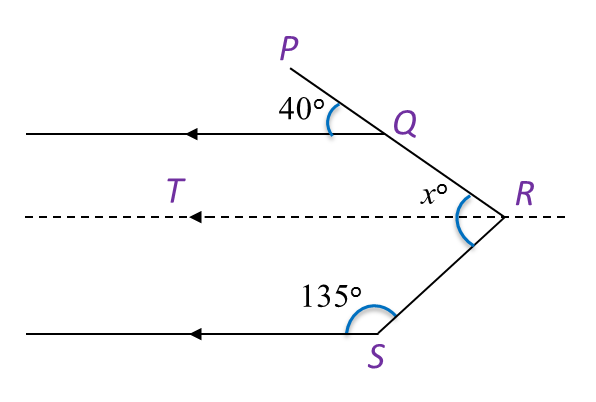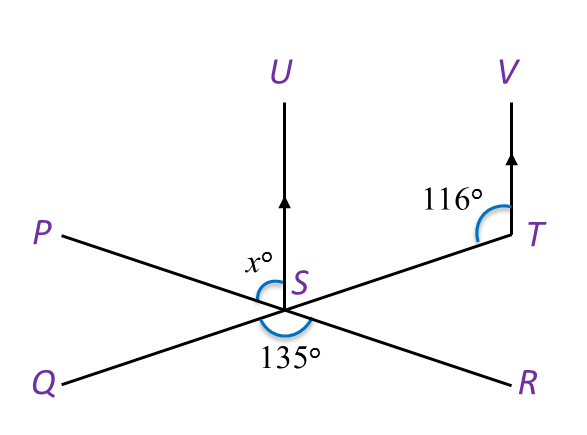# 8.2.2 Lines and Angles, PT3 Practice

 
 
Question 4:
 
In Diagram below, PQR is a straight line. Find the value of x.(adsbygoogle = window.adsbygoogle || []).push({}); Solution:$\begin{array}{l}{x}^{o}=\angle QRT+\angle TRS\\ \angle QRT={40}^{o}\\ \angle TRS={180}^{o}-{135}^{o}\\ \text{}={45}^{o}\\ \text{Hence,}{x}^{o}={40}^{o}+{45}^{o}\\ \text{}{x}^{o}={85}^{o}\\ \text{}x=85\end{array}$  
 
 
 
Question 5:
In Diagram below, find the value of y.Solution:Question 6:
In Diagram below, PSR and QST are straight lines.Find the value of x.

Solution:
$\begin{array}{l}\angle UST+\angle STV={180}^{o}\\ \angle UST={180}^{o}-{116}^{o}={64}^{o}\\ \angle PST=\angle QSR\\ {x}^{o}+\angle UST={135}^{o}\\ {x}^{o}+\angle UST={135}^{o}\\ {x}^{o}={71}^{o}\\ x=71\end{array}$